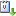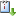Usted está aquí: A proof of two conjectures on Markov Numbers

# A proof of two conjectures on Markov Numbers

Ponente: Li Li
Institución: Oakland University
Tipo de Evento: Investigación
Cuándo 08/09/2020 de 10:00 a 11:00 https://paginas.matem.unam.mx/ocasvCaliCal

La exposición es parte del Online Cluster Algebra Seminar, en cuyo comité organizador participa Daniel Labardini.

A proof of two conjectures on Markov Numbers

A Markov number is a positive integer that is part of a solution $(x,y,z)$, called a Markov triple, to the Markov Diophatine equation $x^2+y^2+z^2=3xyz$. These numbers are related to combinatorics, hyperbolic geometry, approximation theory, and cluster algebras. In Aigner's book "Markov's theorem and 100 years of the uniqueness conjecture'', there are three conjectures asserting an orderings on various subsets of the Markov numbers, namely the constant numerator conjecture, constant denominator conjecture, and constant sum conjecture. The first conjecture was proved in a recent paper by M. Rabideau and R. Schiffler. In this talk, I will explain these three conjectures and present a proof using Ptolemy relations in hyperbolic geometry. This is a joint work with K. Lee, M. Rabideau and R. Schiffler.